# How to calculate time in Excel - time difference, adding / subtracting times

This tutorial focuses on various ways to calculate times in Excel. You will find a few useful formulas to add and subtract times, calculate time difference, or elapsed time, and more.

In the last week's article, we had a close look at the specificities of Excel time format and capabilities of basic time functions. Today, we are going to dive deeper into Excel time calculations and you will learn a few more formulas to efficiently manipulate times in your worksheets.

## How to calculate time difference in Excel (elapsed time)

To begin with, let's see how you can quickly calculate elapsed time in Excel, i.e. find the difference between a beginning time and an ending time. And as is often the case, there is more than one formula to perform time calculations. Which one to choose depends on your dataset and exactly what result you are trying to achieve. So, let's run through all methods, one at a time.

#### Add and subtract time in Excel with a special tool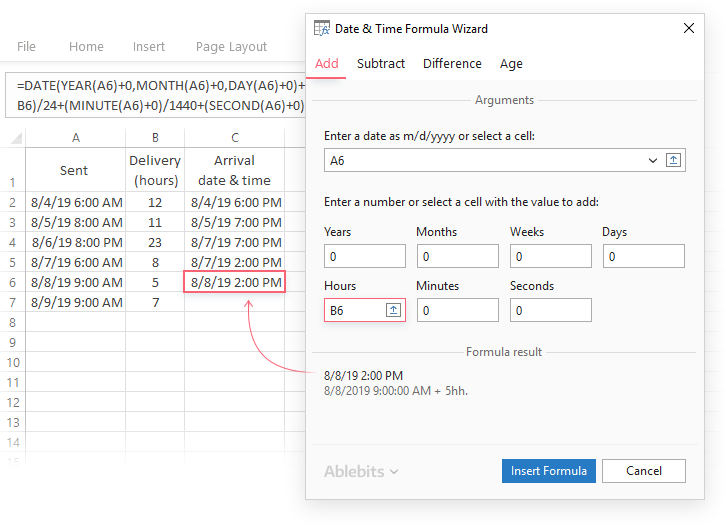Read more

#### Easily find difference between two dates in Excel

##### Get the result as a ready-made formula in years, months, weeks, or days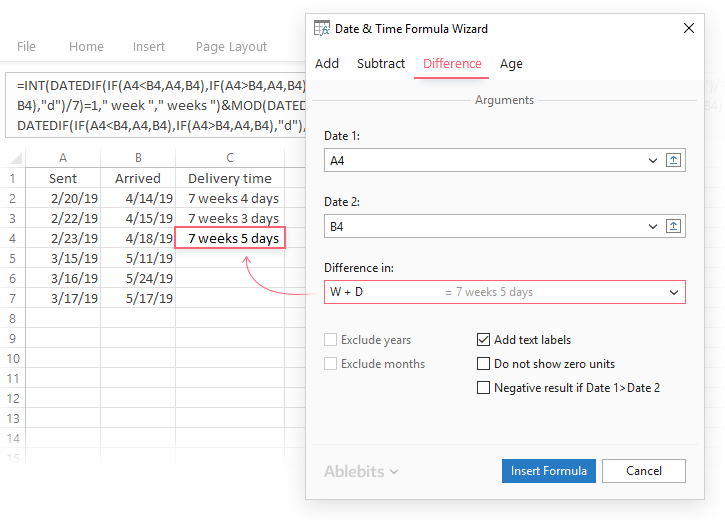Read more

#### Calculate age in Excel on the fly

##### And get a custom-tailored formula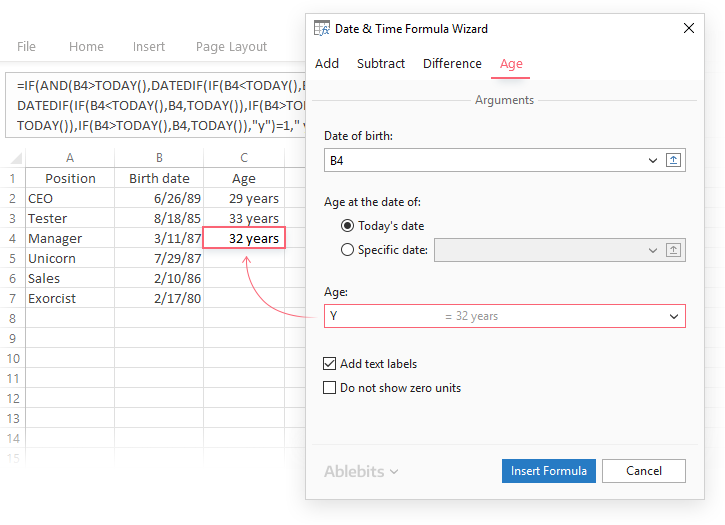Read more

#### Formula 1. Subtract one time from the other

As you probably know, times in Excel are usual decimal numbers formatted to look like times. And because they are numbers, you can add and subtract times just as any other numerical values.

The simplest and most obvious Excel formula to calculate time difference is this:

=End time - Start time

Depending on you data structure, the actual time difference formula may take various shapes, for example:

 Formula Explanation `=A2-B2` Calculates the difference between the time values in cells A2 and B2. `=TIMEVALUE("8:30 PM") - TIMEVALUE("6:40 AM")` Calculates the difference between the specified times. `=TIME(HOUR(A2), MINUTE(A2), SECOND(A2)) - TIME(HOUR(B2), MINUTE(B2), SECOND(B2))` Calculates the time difference between values in cells A2 and B2 ignoring the date difference, when the cells contain both the date and time values.

Remembering that in the internal Excel system, times are represented by fractional parts of decimal numbers, you are likely to get the results similar to this: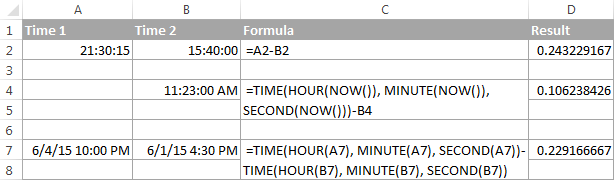The decimals in column D are perfectly true but not very meaningful. To make them more informative, you can apply custom time formatting with one of the following codes:

 Time code Explanation h Elapsed hours, display as 4. h:mm Elapsed hours and minutes, display as 4:10. h:mm:ss Elapsed hours, minutes and seconds, display as 4:10:20.

To apply the custom time format, click Ctrl + 1 to open the Format Cells dialog, select Custom from the Category list and type the time codes in the Type box. Please see Creating a custom time format in Excel for the detailed steps.

And now, let's see how our time difference formula and time codes work in real worksheets. With Start times residing in column A and End times in column B, you can copy the following formula in columns C though E:

`=\$B2-\$A2`

The elapsed time is displayed differently depending on the time format applied to the column: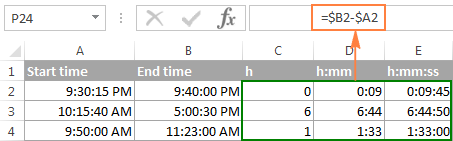Note. If the elapsed time is displayed as hash marks (#####), then either a cell with the formula is not wide enough to fit the time or the result of your time calculations is a negative value.

#### Formula 2. Calculating time difference with the TEXT function

Another simple technique to calculate the duration between two times in Excel is using the TEXT function:

• Calculate hours between two times: `=TEXT(B2-A2, "h")`
• Return hours and minutes between 2 times: `=TEXT(B2-A2, "h:mm")`
• Return hours, minutes and seconds between 2 times: `=TEXT(B2-A2, "h:mm:ss")`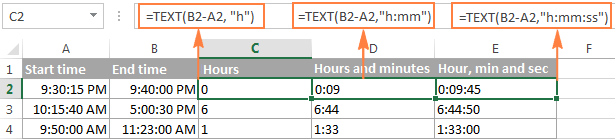Notes:

• The value returned by the TEXT function is always text. Please notice the left alignment of text values in columns C:E in the screenshot above. In certain scenarios, this might be a significant limitation because you won't be able to use the returned "text times" in other calculations.
• If the result is a negative number, the TEXT formula returns the #VALUE! error.

#### Formula 3. Count hours, minutes or seconds between two times

To get the time difference in a single time unit (hours ,minutes or seconds), you can perform the following calculations.

Calculate hours between two times:

To present the difference between two times as a decimal number, use this formula:

=(End time - Start time) * 24

Supposing that your start time is in A2 and end time in B2, you can use a simple equation B2-A2 to calculate the difference between two times, and then multiply it by 24, which is the number of hours in one day:

`=(B2-A2) * 24`

To get the number of complete hours, use the INT function to round the result down to the nearest integer:

`=INT((B2-A2) * 24)`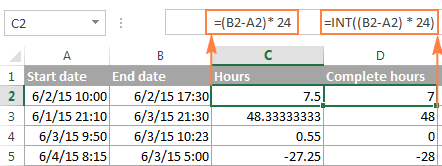Total minutes between two times:

To calculate the minutes between two times, multiply the time difference by 1440, which is the number of minutes in one day (24 hours * 60 minutes = 1440).

=(End time - Start time) * 1440

As demonstrated in the following screenshot, the formula can return both positive and negative values, the latter occur when the end time is less than the start time, like in row 5:

`=(B2-A2)*1440`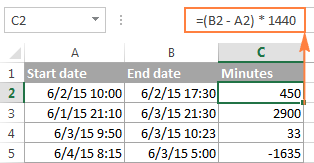Total seconds between times:

To get the total seconds between two times, you multiply the time difference by 86400, which is the number of seconds in one day (24 hours * 60 minutes * 60 seconds = 86400).

=(End time - Start time) * 86400

In our example, the formula is as follows:

`=(B2-A2)* 86400`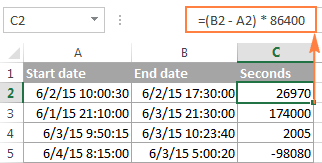Note. For the results to display correctly, the General format should be applied to the cells with your time difference formula.

#### Formula 4. Calculate difference in one time unit ignoring others

To find the difference between 2 times in a certain time unit, ignoring the others, use one of the following functions.

• Difference in hours, ignoring minutes and seconds:

`=HOUR(B2-A2)`

• Difference in minutes, ignoring hours and seconds:

`=MINUTE(B2-A2)`

• Difference in seconds, ignoring hours and minutes:

`=SECOND(B2-A2)`

When using Excel's HOUR, MINUTE and SECOND functions, please remember that the result cannot exceed 24 for hours and 60 for minutes and seconds.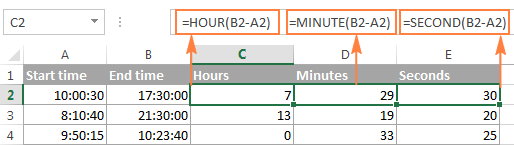Note. If the end time is less than the start time (i.e. the result of the formula is a negative number), the #NUM! error is returned.

#### Formula 5. Calculate elapsed time from a start time to now

In order to calculate how much time has elapsed since the start time to now, you simply use the NOW function to return today's date and the current time, and then subtract the start date and time from it.

Supposing that the beginning date and time is in call A2, the formula `=NOW()-A2` returns the following results, provided you've applied an appropriate time format to column B, h:mm in this example: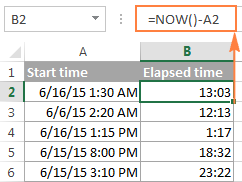In case the elapsed time exceeds 24 hours, use one of these time formats, for example d "days" h:mm:ss like in the following screenshot: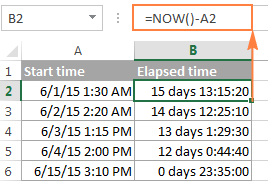If your starting points contain only time values without dates, you need to use the TIME function to calculate the elapsed time correctly. For example, the following formula returns the time elapsed since the time value in cell A2 up to now:

`=TIME(HOUR(NOW()), MINUTE(NOW()), SECOND(NOW())) - A2`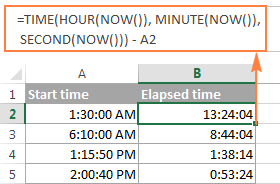Note. The elapsed time is not updated in real-time, it refreshes only when the workbook is reopened or recalculated. To force the formula to update, press either Shift + F9 to recalculate the active spreadsheet or hit F9 to recalculate all open workbooks.

#### Formula 5. Display time difference as "XX days, XX hours, XX minutes and XX seconds"

This is probably the most user-friendly formula to calculate time difference in Excel. You use the HOUR, MINUTE and SECOND functions to return corresponding time units and the INT function to compute the difference in days. And then, you concatenate all these functions in a single formula along with the text labels:

`=INT(B2-A2) & " days, " & HOUR(B2-A2) & " hours, " & MINUTE(B2-A2) & " minutes and " & SECOND(B2-A2) & " seconds"`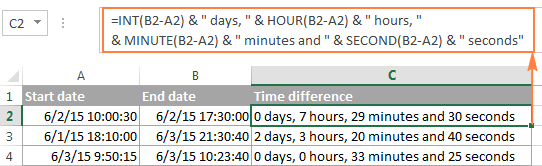To instruct your Excel time difference formula to hide zero values, embed four IF functions into it:

`=IF(INT(B2-A2)>0, INT(B2-A2) & " days, ","") & IF(HOUR(B2-A2)>0, HOUR(B2-A2) & " hours, ","") & IF(MINUTE(B2-A2)>0, MINUTE(B2-A2) & " minutes and ","") & IF(SECOND(B2-A2)>0, SECOND(B2-A2) & " seconds","")`

The syntax may seem excessively complicated, but it works :)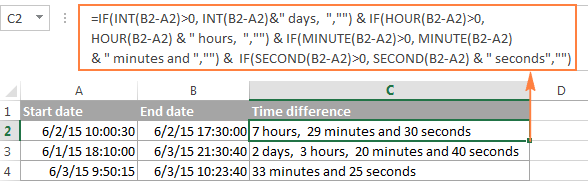Alternatively, you can calculate time difference by simply subtracting the start time from the end time (e.g. `=B2-A2`), and then apply the following time format to the cell:

d "days," h "hours," m "minutes and" s "seconds"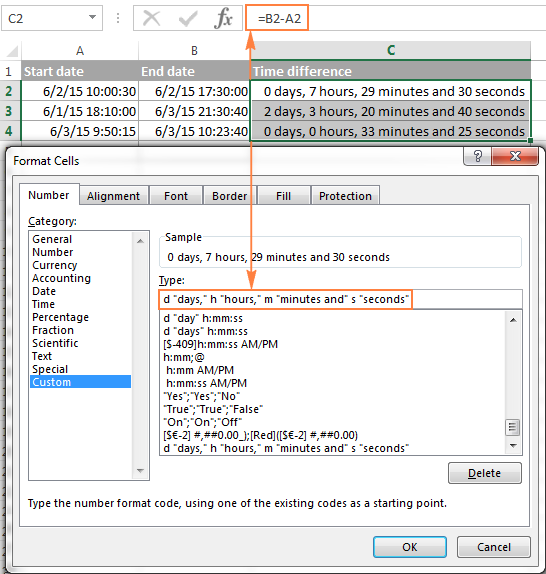An advantage of this approach is that your result would be a normal time value that you could use in other time calculations, while the result of the complex formula discussed above is a text value. A drawback is that the custom time format cannot distinguish between zero and non-zero values and ignore the latter. To display the result in other formats, please see Custom formats for time intervals over 24 hours.

## How to calculate and display negative times in Excel

When calculating the time difference in Excel, you may sometimes get the result as ###### error because the difference is a negative time. But is there a way to show negative times properly in Excel? Of course, there is a way, and even more than one :)

#### Method 1. Change Excel Date System to 1904 date system

The fastest and easiest way to display negative time normally (with a minus sign) is switching to the 1904 date system. To do this, click File > Options > Advanced, scroll down to the When calculating this workbook section and put a tick in the Use 1904 date system box.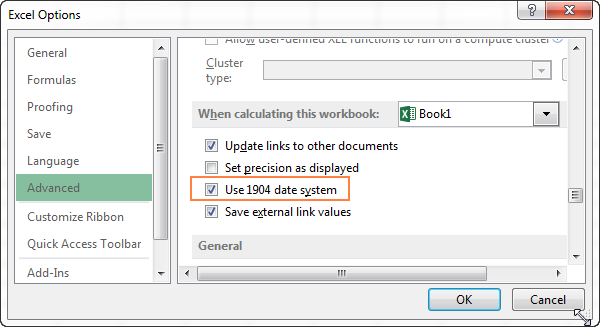Click OK to save the new settings, and from now on negative times will be displayed correctly, like negative numbers: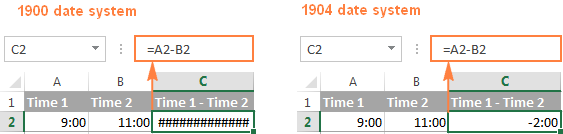#### Method 2. Calculate negative time in Excel with formulas

Is changing Excel's default Date System is not an option, then you can force negative times to display properly using one of the following formulas:

`=IF(A2-B2>0, A2-B2, "-" & TEXT(ABS(A2-B2),"h:mm"))`

`=IF(A2-B2>0, A2-B2, TEXT(ABS(A2-B2),"-h:mm"))`

Both formulas check if the time difference (A2-B2) is greater than 0, and if it is they return that difference. If the time difference is less than zero, the first formula calculates the absolute difference and concatenates the minus sign. The second formula yields exactly the same result by using a negative time format "-h::mm".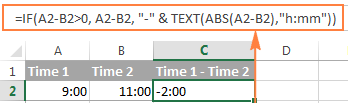Note. Please keep in mind that unlike the first method that treats negative times as negative numeric values, the result of the TEXT function is always a text string that cannot be used in calculations or other formulas.

## Adding and subtracting time in Excel

Basically, there are 2 ways to add and subtract time in Excel:

• Using the TIME function
• Using arithmetic calculations based on the number of hours (24), minutes (1440) and seconds (86400) in one day

The `TIME(hour, minute, second)` function makes Excel time calculations really easy, however it does not allow adding or subtracting more than 23 hours, or 59 minutes, or 59 seconds. If you are working with bigger time intervals, then use one of the arithmetic calculations demonstrated below.

### How to add or subtract hours to time in Excel

To add hours to a given time in Excel, you can use one the following formulas.

TIME function to add under 24 hours

=Start time + TIME(N hours, 0, 0)

For example, if your start time is in cell A2, and you want to add 2 hours to it, the formula is as follows:

`=A2 + TIME(2, 0, 0)`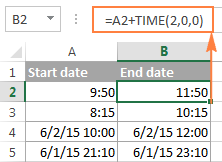Note. If you try adding more than 23 hours with the TIME function, the specified hours will be divided by 24 and the remainder will be added to the start time value. For example, if you try to add 25 hours to "6/2/2015 10:00 AM" (cell A4) using the formula `=A4 + TIME(25, 0, 0)`, the result will be "06/02/2015 11:00", i.e. A4 + 1 hour.

Formula to add any number of hours (under or over 24 hours)

The following formula has no limitations to the number of hours you want to add:

= Start time + (N hours / 24)

For example, to add 28 hours to the start time in cell A2, enter the following formula:

`=A2 + (28/24)`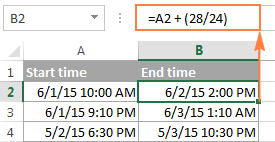To subtract hours from a given time, you use analogous formulas, and just replace "+" with the minus sign:

For example, to subtract 3 hours from the time in cell A2, either of the following formulas will do:

`=A2-(3/24)`

`=A2-TIME(3,0,0)`

To subtract more than 23 hours, use the first one.

### How to add / subtract minutes to time in Excel

To add minutes to a given time, employ the same techniques that we've just used for adding hours.

To add or subtract under 60 minutes

Use the TIME function and supply the minutes you want to add or subtract in the second argument:

=Start time + TIME(0, N minutes, 0)

And here are a couple of real-life formulas to calculate minutes in Excel:

To add 20 minutes to the time in A2: =A2 + TIME(0,20,0)

To subtract 30 minutes from the time in A2: =A2 - TIME(0,30,0)

To add or subtract over 60 minutes

In your calculation, divide the number of minutes by 1440, which is the number of minutes in a day, and add the quotient to the start time:

=Start time + (N minutes / 1440)

To subtract minutes from time, simply replace plus with the minus sign. For example:

To add 200 minutes: `=A2 + (200/1440)`

To subtract 300 minutes: `=A2 -(300/1440)`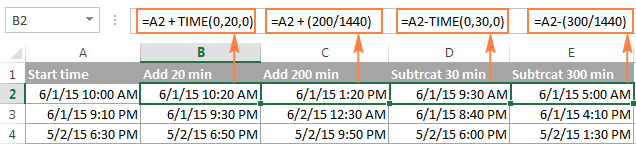### How to add / subtract seconds to a given time

Second calculations in Excel are done in a similar fashion.

To add under 60 seconds to a given time, you can use the TIME function:

=Start time + TIME(0, 0, N seconds)

To add more than 59 seconds, use the following formula:

=Start time + (N seconds / 86400)

To subtract seconds, utilize the same formulas with the minus sign (-) instead of plus (+).

In your Excel worksheets, the formulas may look similar to these:

To add 30 seconds to A2: `=A2 + TIME(0,0,31)`

To add 1200 seconds to A2: `=A2 + (1200/86400)`

To subtract 40 seconds from A2: `=A2 - TIME(0,0,40)`

To subtract 900 seconds from A2: `=A2 - (900/86400)`

## How to sum time in Excel

The Excel sum time formula is the usual SUM function, and applying the proper time format to the result is what does the trick.

Supposing you have a few project times in column B and you want to add them up. You write a simple SUM formula like `=SUM(B2:B4)` and get the result in the default format, like hh:mm:ss.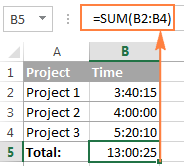In some cases the default time format works just fine, but sometimes you may want more, for example to display the total time as minutes and seconds, or seconds only. The good news is that no other calculations are required, all you have to do is apply custom time format to the cell with the SUM formula.

Right click the cell and select Format Cells in the context menu, or press Ctrl + 1 to open the Format Cells dialog box. Select Custom from the Category list and type one of the following time formats in the Type box:

• To display total time as minutes and seconds: [m]:ss
• To display total time as seconds: [ss]

The result will look as follows: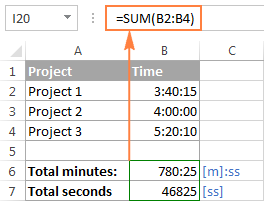### How to sum over 24 hours in Excel

In order to add up more than 24 hours, you use the same SUM formula as discussed above, and apply one of the following time formats to the cell:

 Format Displays as Explanation [h]:mm 30:10 Hours and minutes [h]:mm:ss 30:10:20 Hours, minutes and seconds [h] "hours", mm "minutes", ss "seconds" 30 hours, 10 minutes, 20 seconds d h:mm:ss 1 06:10:20 Days, hours, minutes and seconds d "day" h:mm:ss 1 day 06:10:20 d "day," h "hours," m "minutes and" s "seconds" 1 day, 6 hours, 10 minutes and 20 seconds

To see how these custom time formats may look like in your Excel worksheet, please have a look at the screenshot below, where the same SUM formula is entered in cells A9 to A13:

`=SUM(\$B\$2:\$B\$4)`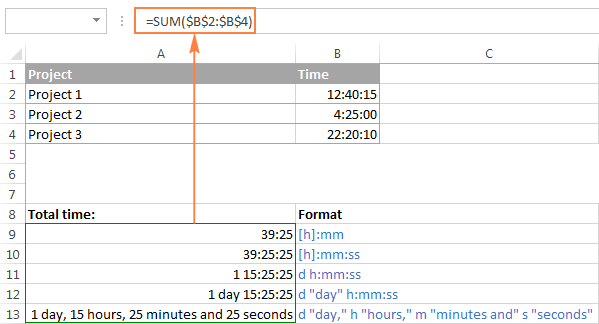Note. The above custom time formats work for positive values only. If the result of your time calculations is a negative number, e.g. when you are subtracting a bigger time from a smaller time, the result will be displayed as #####. To display negative times differently, please see custom format for negative time values.

Also, please keep in mind that the time format applied to a cell changes only the display presentation without changing the cell's value. For example, in the screenshot above, cell A13 looks like text, but in fact it's a usual time value, which is stored as a decimal in the internal Excel system. Meaning, you are free to refer to that cell in other formulas and calculations.

To have a closer look at the time formulas discussed in this article, feel free to download our Sample Workbook with Time Calculations.

## Date & Time Formula Wizard - quick way to calculate times in Excel

Now that you know a bunch of different formulas to add and subtract times in Excel, let me show you the tool that can do it all. Okay, almost all :)

Here comes Ablebit's Date & Time Formula Wizard for Excel:In the Date & Time Wizard dialog window, you switch to the Add or Subtract tab, depending on which operation you want to perform, and do the following:

• Click the Show time fields link in the left part of the window.
• Supply values or cell references for the formula arguments. As you fill in the argument boxes, the wizard builds the formula in the selected cell.
• When finished, click the Insert Formula

That's it! For example, this is how you can add the specified number of hours, minutes and seconds to the time in A4: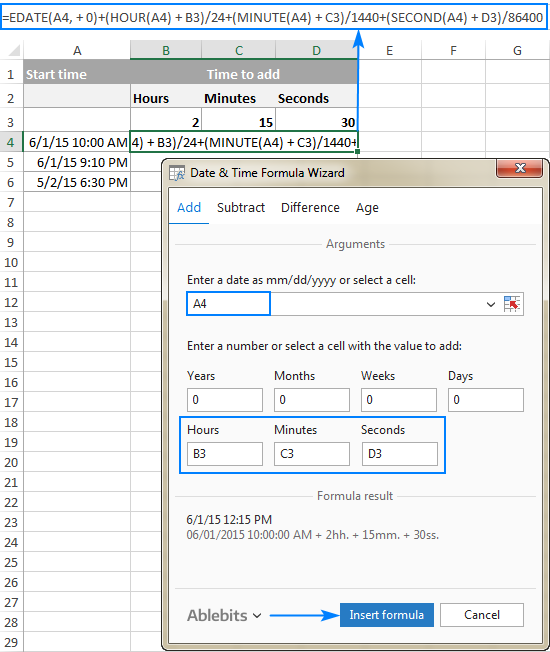If you need to copy the formula to other cells, fix all references except the cell containing the original time (A4) with the \$ sign like shown in the screenshot below (by default, the wizard always uses relative references). Then double-click the fill handle to copy the formula down the column and you are good to go!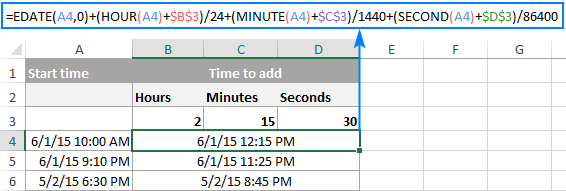Besides time calculations, the wizard can also add and subtract dates, get the difference between two dates, and calculate age from the birthdate.

If you are curious to try this tool in your own worksheets, you are welcome to download the 14-day trial version of our Ultimate Suite which includes this as well as 60 more time saving add-ins for Excel.

This is how you calculate time in Excel worksheets. To learn other ways to manipulate dates and times in Excel, I encourage you to check out the resources at the end of this article. I thank you for reading and hope to see you on our blog next week!